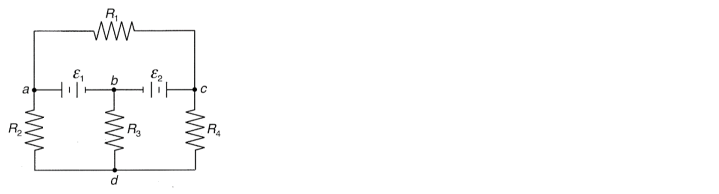# Problem: For the circuit below, determine all the currents. Assume ε1 = 10 V, ε2 = 15 V, R 1 = R 3 = 40 Ω, R 2 = 60 Ω, R 4 = 100 Ω.

###### FREE Expert Solution
88% (206 ratings)
###### Problem Details

For the circuit below, determine all the currents. Assume ε1 = 10 V, ε2 = 15 V, R = R = 40 Ω, R 2 = 60 Ω, R 4 = 100 Ω.